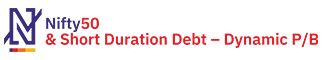• As on ,
• All Prices are in INR## NIFTY50 & Short Duration Debt – Dynamic P/B Index capture the performance of portfolio where asset is allocated among NIFTY 50 TR Index (equity component), NIFTY Short duration debt index (debt component), NIFTY 50 Arbitrage, and CBLO based on current Price-Book ratio (P/B) of NIFTY 50.

### Highlights

• The indices have a base date of  1st April, 2005 and a base value of 1000
• Asset allocation is based on the comparison of current Price-Book ratio (P/B) with the average, maximum, minimum Price-Book ratio (P/B) of NIFTY 50 in previous 7 years
• Maximum allocation to equity component is 80% and minimum allocation is 65% including arbitrage
• Maximum allocation to debt component is 35% and minimum allocation is 20%
• The index is rebalanced on a monthly basis
• Weights of the equity and fixed income index can drift between monthly reset dates due to underlying asset price movement